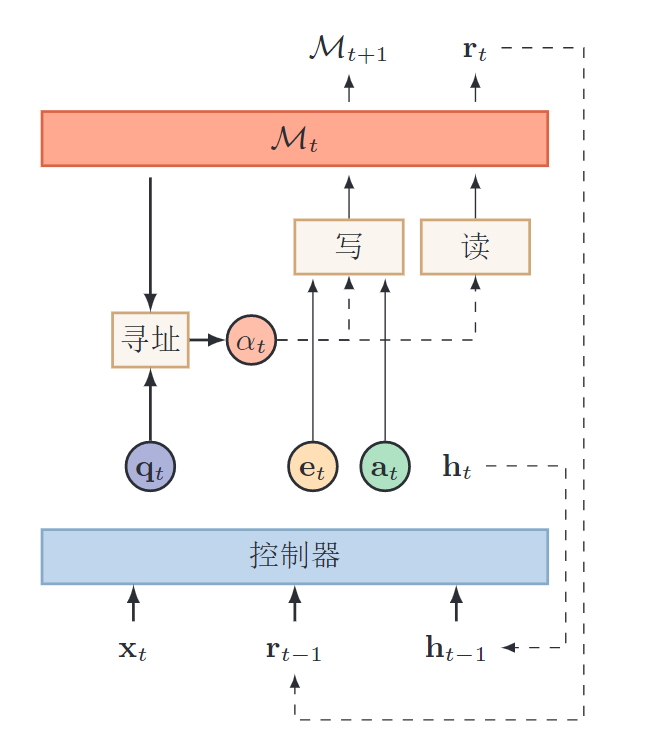# 注意力机制系统介绍

## 问题背景

• 信息选择：聚焦式自上而下地选择重要信息，过滤掉无关的信息。注意力机制
• 外部记忆 ： 优化神经网络的记忆结构，使用额外的外部记忆，来提高网络的存储信息的容量。 记忆力机制

## 注意力

• 噪音很多，依然可以听到朋友谈话的内容
• 没有关注背景声音，但是突然有人叫自己（重要信息），依然会马上注意到

## 普通注意力机制

$X_{1:N} = [\mathbf{x}_1, \cdots, \mathbf{x}_N]$， 问题$\mathbf{q}$。 要从$X$中选择一些和任务相关的信息输入给神经网络。

$\alpha_i$ : 选择第$i$个信息的概率，也称为注意力分布$z$表示被选择信息的索引位置 $\alpha_i = p(z = i \mid X, \mathbf{q}) = \rm{softmax}\left(s(\mathbf{x}_i, \mathbf{q})\right) = \frac{\exp\left(s(\mathbf{x}_i, \mathbf{q})\right)}{\sum_{j=1}^N \exp\left(s(\mathbf{x}_j, \mathbf{q})\right)}$

NMT里面三种score打分函数 : $\color{blue}{\rm{score}(h_t, \bar h_s)} = \begin{cases} h_t^T \bar h_s & \text{dot} \\ h_t^T W_a \bar h_s & \text{general} \\ v_a^T \tanh (W_a [h_t; \bar h_s]) & \text{concat} \\ \end{cases}$ 加性模型 $s(\mathbf{x}_i, \mathbf{q}) = v^T\rm{tanh} (W\mathbf{x}_i + U\mathbf{q})$ 点击模型 $s(\mathbf{x}_i, \mathbf{q}) = \mathbf{x}_i^T \mathbf{q}$ 计算注意力

Soft Attention 是对所有的信息进行加权求和。Hard Attention是选择最大信息的那一个。

## 应用与优点

• 编码向量容量瓶颈问题：所有信息都需要保存在编码向量中
• 长距离依赖问题：长距离信息传递时，信息会丢失

• 解码过程中每一步都直接访问源语言所有位置上的信息。无需让所有信息都通过编码向量进行传递。
• 缩短了信息的传递距离。源语言的信息可以直接传递到解码过程中的每一步

# 注意力机制变体

## 多头注意力

Multi-head Attention利用多个查询$\mathbf{q}_{1:M}={\mathbf{q}_1, \cdots, \mathbf{q}_M}$来并行地从输入信息中选取多个信息。每个注意力关注输入信息的不同部分。比如Attention Is All You Need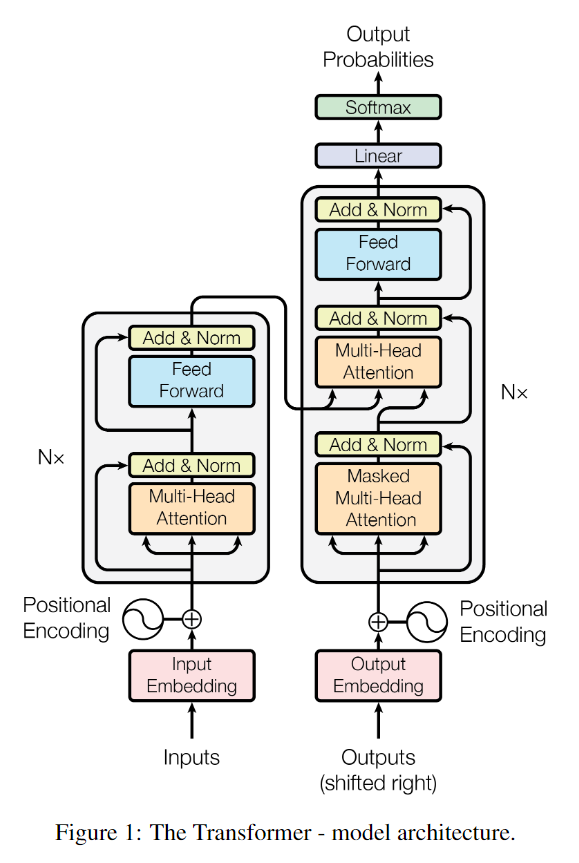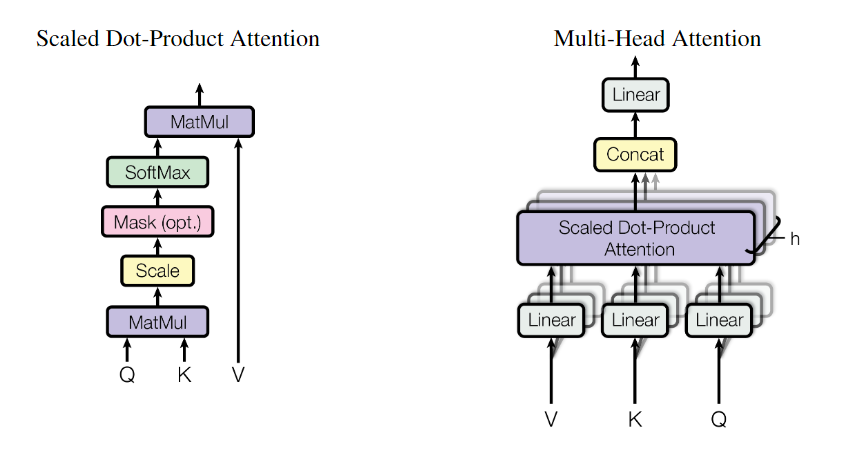• 选取最高概率的输入信息
• 在注意力分布上随机采样

## 指针网络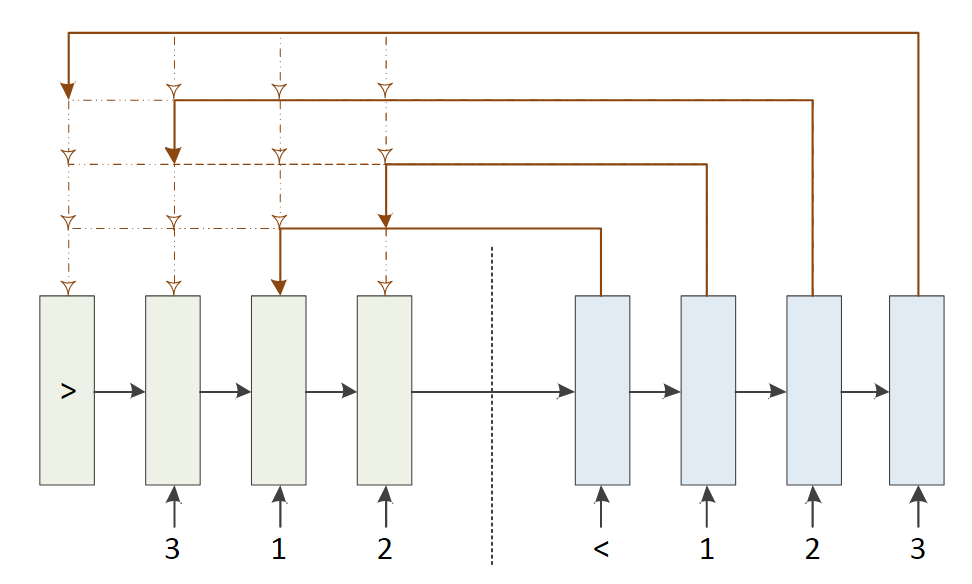$p(c_i \mid c_{1:i-1}, \mathbf x_{1:n}) = \rm{softmax}(s_{i,j})$

# 各种注意力计算模型

## 注意力的本质

$k$$d$维的特征向量$\mathbf h_i \;(i \in [1,k])$，想要整合这k个特征向量的信息。得到一个向量$\mathbf h^*$，一般也是d维。

• 简单粗暴：对k个向量求平均。当然不合理啦。
• 加权平均：$\mathbf h^* = \sum_{i=1}^k \alpha_i \mathbf h_i$合理

• 针对每个$\mathbf h_i$， 计算出一个得分$s_i$$h_i$$q$越相关，得分越高。
• $\alpha_i = \rm{softmax}(s_i)$

$s_i = \rm{score}(\mathbf h_i, \mathbf q)$

• Local-based Attention ，没有外部的关注对象，自己关注自己。
• General Attention， 有外部的关注对象，直接乘积，全连接层。
• Concatenation-based Attention， 有关注的对象，先concat或相加再过连接层。

## Local-based

$\mathbf h^* = \sum_{i=1}^n s_i \cdot \mathbf h_i$

a是激活函数sigmoid, tanh, relu, maxouty=x（无激活函数）。

1 一个得分简单求法

A Context-Aware Attention Network For Interactive Question Answering

Dynamic Attention Deep Model for Article Recommendation by Learning Human Editors’ Demonstration

CAN for QA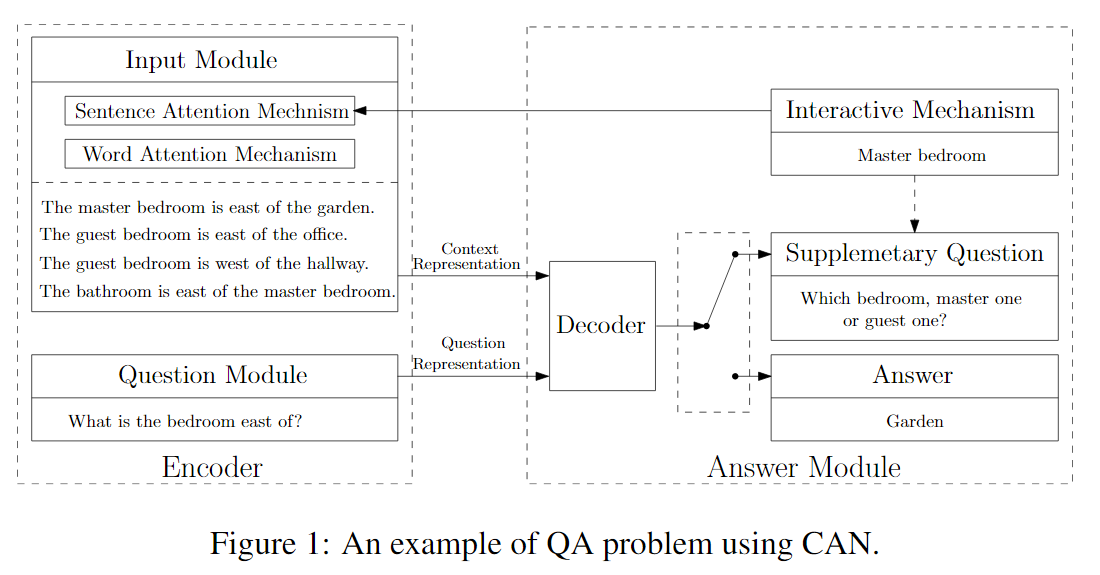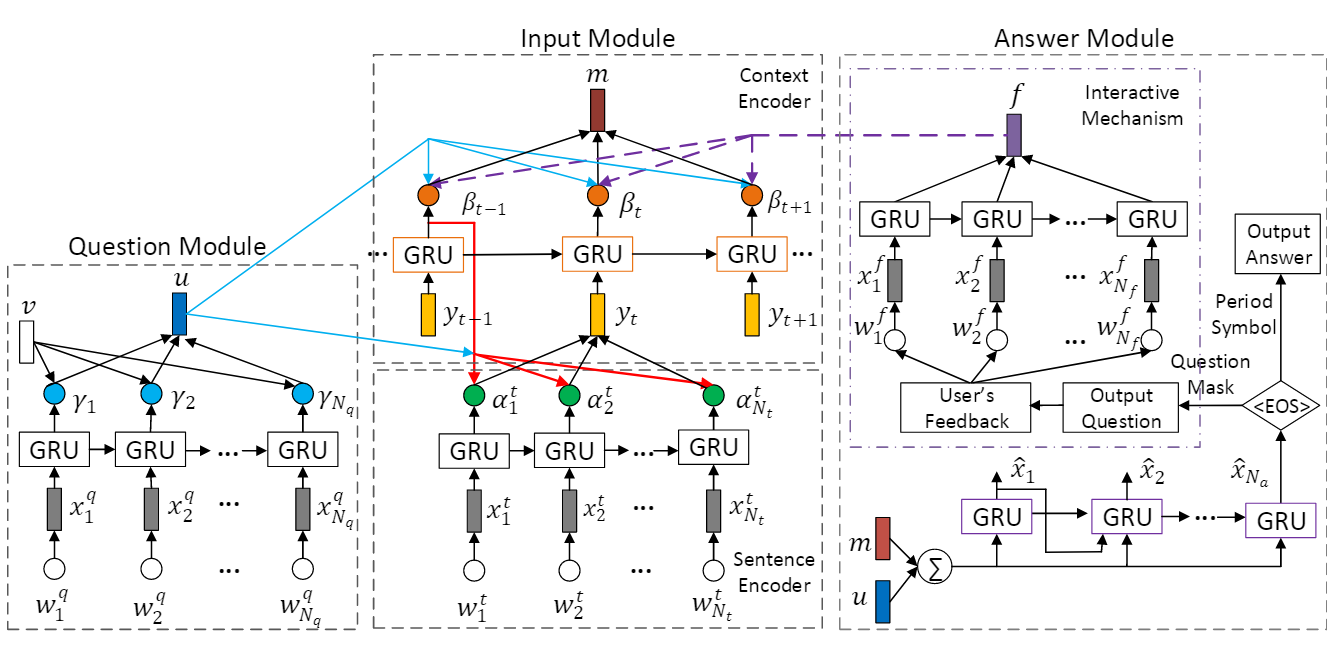Dynamic Attention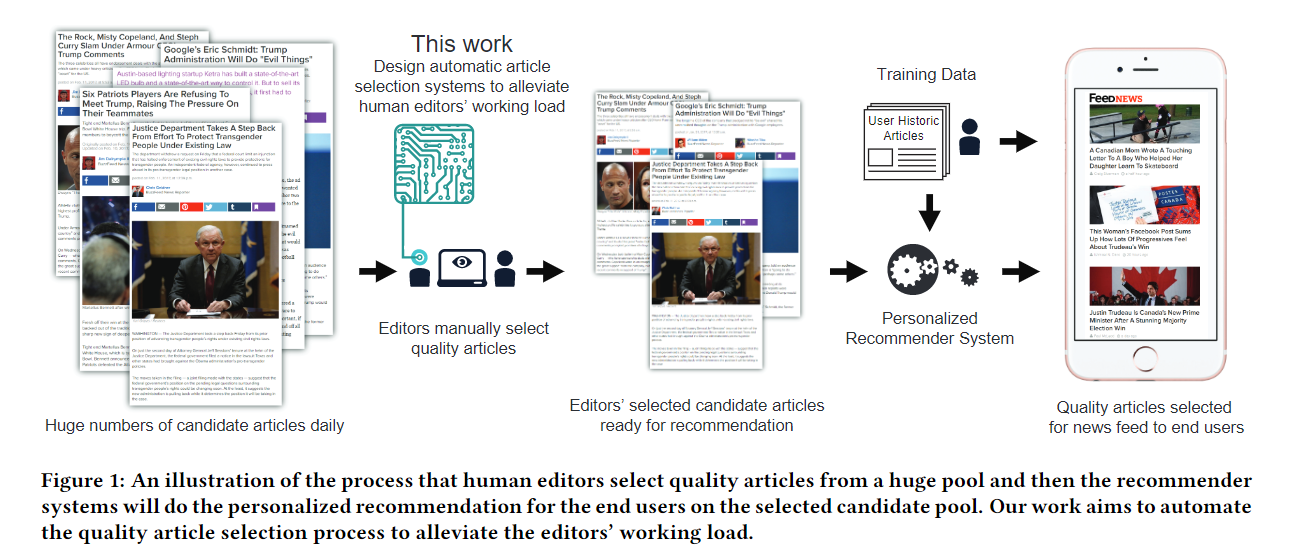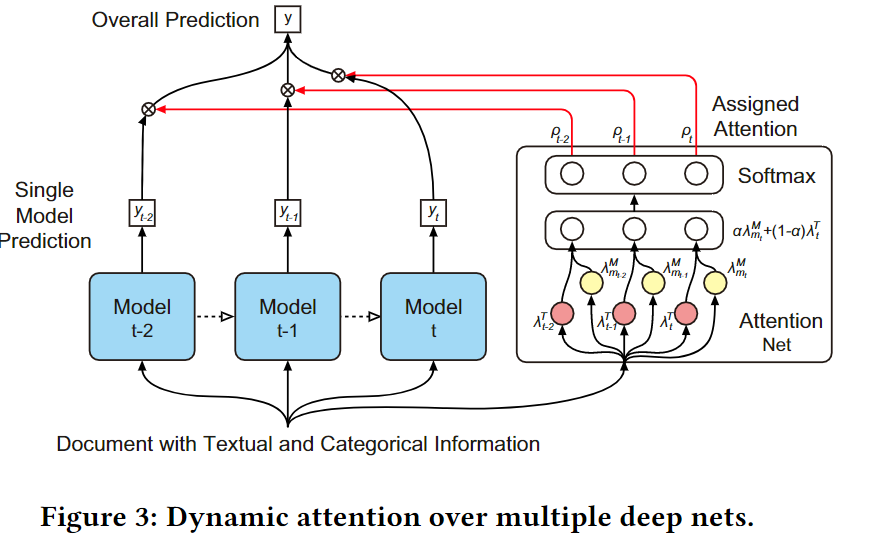## Concatenation-based

Attentive Collaborative Filtering Multimedia Recommendation with Item- and Component-Level Attention_sigir17

Item-Level Attention。可以看到需要加什么Attention，直接向公式里面一加就可以了。 $a(i, l) = w_1^T \phi(W_{1u} \mathbf u_i + W_{1v} \mathbf v_l + W_{1p} \mathbf p_l + W_{1x} \mathbf {\bar x}_l + \mathbf b_1) + \mathbf c_1$

$\alpha(i, l) = \frac {\exp (a(i, l))} {\sum_{n \in R(i)} \exp (a(i, n))}$

## 多层Attention

$m$个句子，每个句子有$k$个词语。

Word-level Attention

Sentence-level Attention

$m$个句子，每个句子是一个句子向量$\mathbf s_i$。 可以再次Attention，得到文档的向量表达$\mathbf d$， 也可以得到每个句子的权值$\alpha_i$

1. 文章摘要生成

Leveraging Contextual Sentence Relations for Extractive Summarization Using a Neural Attention Model_SIGIR2017

• 第一层：Local-based Attention， 生成每个句子的vector
• 第二层：当前句子作为中心，2n+1个句子。输入RNN（不明白）。将中心句子作为attention，来编码上下文。通过上下文对中心句子进行打分。作为该句子对整个文本的重要性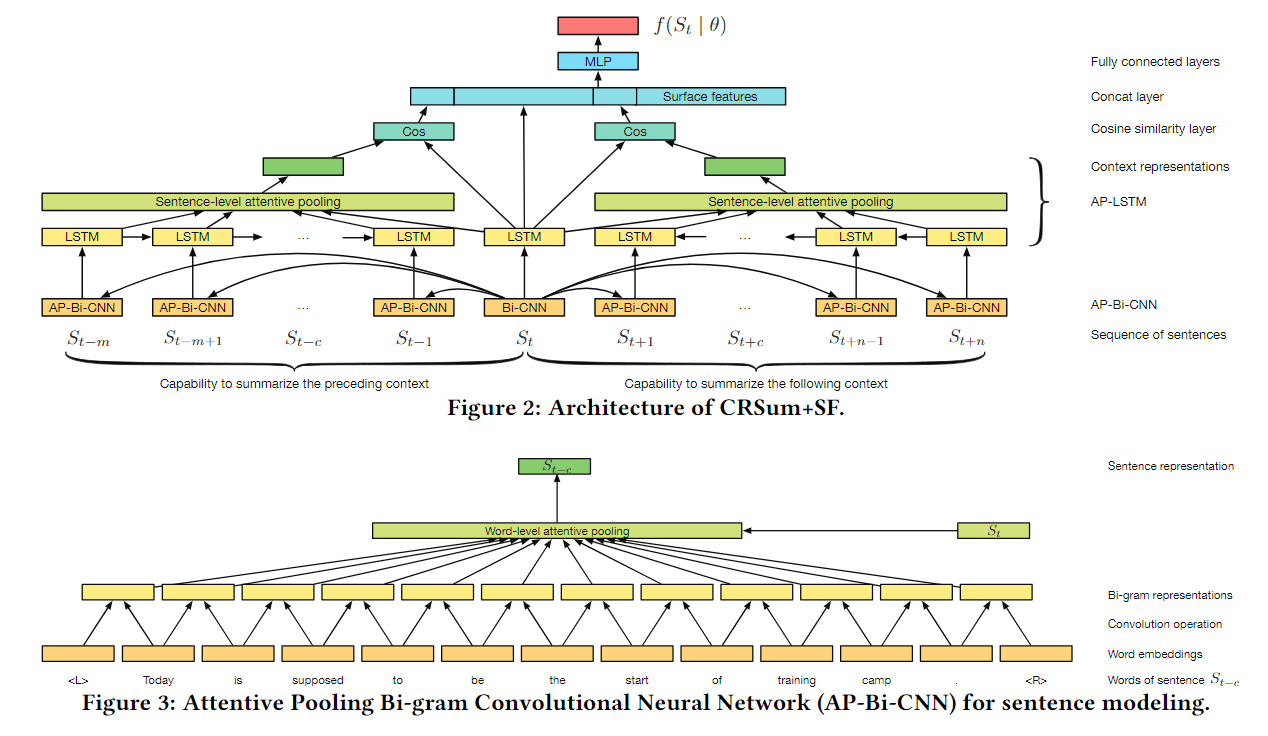## CAN的实时问答

A Context-Aware Attention Network For Interactive Question Answering

• Period Symbol ：是正确答案，直接输出
• Question Mask： 输出是一个问题，要继续问用户相应的信息

$\beta_t = \rm{softmax}(\mathbf u^T \mathbf s_t + \mathbf r^T \mathbf s_t)$